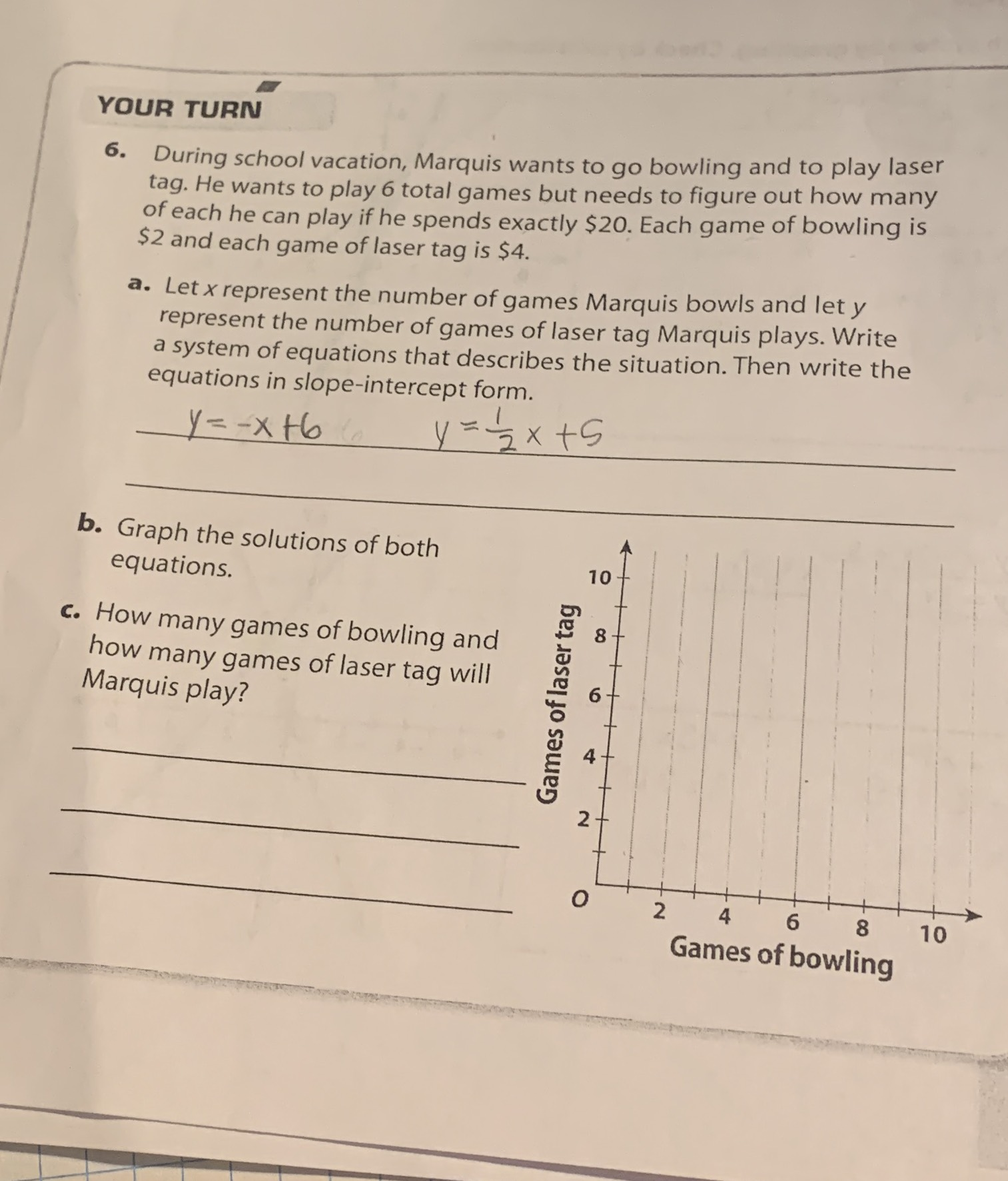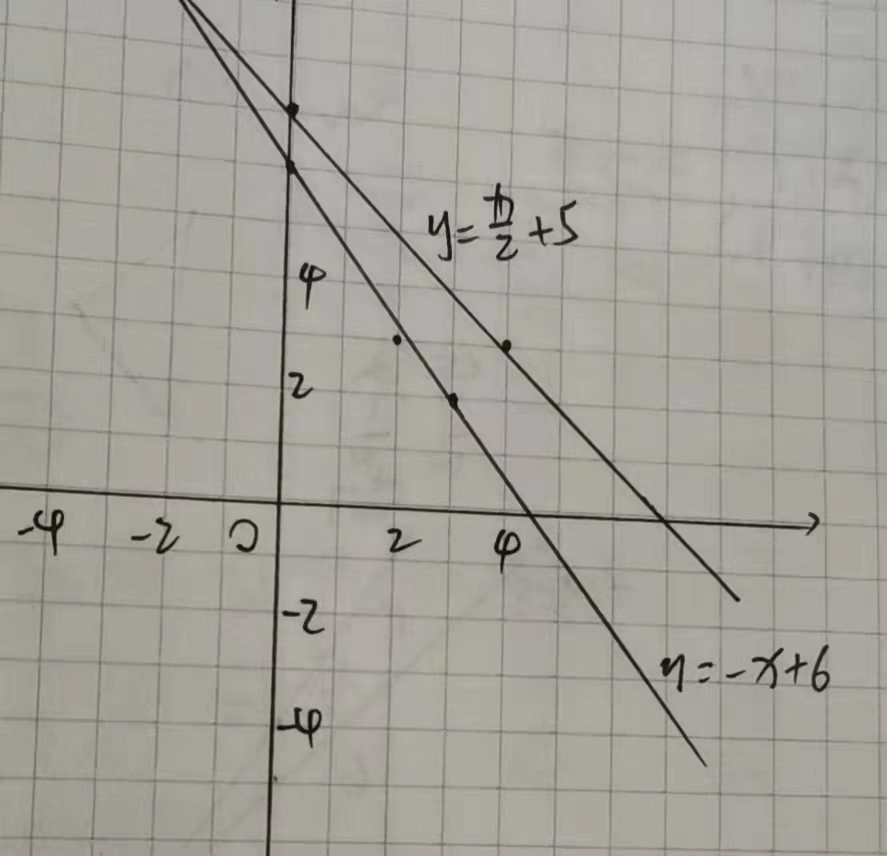### ¿Todavía tienes preguntas de matemáticas?

Pregunte a nuestros tutores expertos
Algebra
Pregunta6. During school vacation, Marquis wants to go bowling and to play laser tag. He wants to play $$6$$ total games but needs to figure out how many of each he can play if he spends exactly $$\ 20$$ . Each game of bowling is $$\ 2$$ and each game of laser tag is $$\ 4$$ .

a. Let $$x$$ represent the number of games Marquis bowls and let $$y$$ represent the number of games of laser tag Marquis plays. Write a system of equations that describes the situation. Then write the equations in slope-intercept form.

b. Graph the solutions of both equations.

c. How many games of bowling and how many games of laser tag will Marquis play?